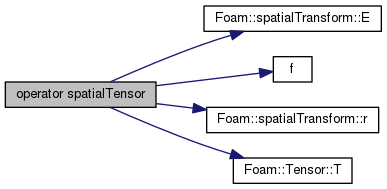The OpenFOAM Foundation
spatialTransform::transpose Class Reference

Wrapper-class to provide transpose functions and operators. More...

## Public Member Functions

transpose (const spatialTransform &X)
Construct from a spatialTransform. More...

operator spatialTensor () const
Return the transpose transformation tensor ^A{X^*}_B. More...

spatialVector operator & (const spatialVector &f) const
Transpose transform dual f: ^A{X^*}_B & f. More...

## Detailed Description

Wrapper-class to provide transpose functions and operators.

Definition at line 88 of file spatialTransform.H.

## ◆ transpose()

 transpose ( const spatialTransform & X )
inline

Construct from a spatialTransform.

Definition at line 63 of file spatialTransformI.H.

## ◆ operator spatialTensor()

 operator spatialTensor ( ) const
inline

Return the transpose transformation tensor ^A{X^*}_B.

X^T

Definition at line 179 of file spatialTransformI.H.

References spatialTransform::E(), f(), spatialTransform::r(), Tensor< Cmpt >::T(), and Foam::Zero.

Here is the call graph for this function:## ◆ operator &()

 spatialVector operator& ( const spatialVector & f ) const
inline

Transpose transform dual f: ^A{X^*}_B & f.

X^T . f = (E^T . fl + r ^ E^T . fw, E^T . fl)

The documentation for this class was generated from the following files: